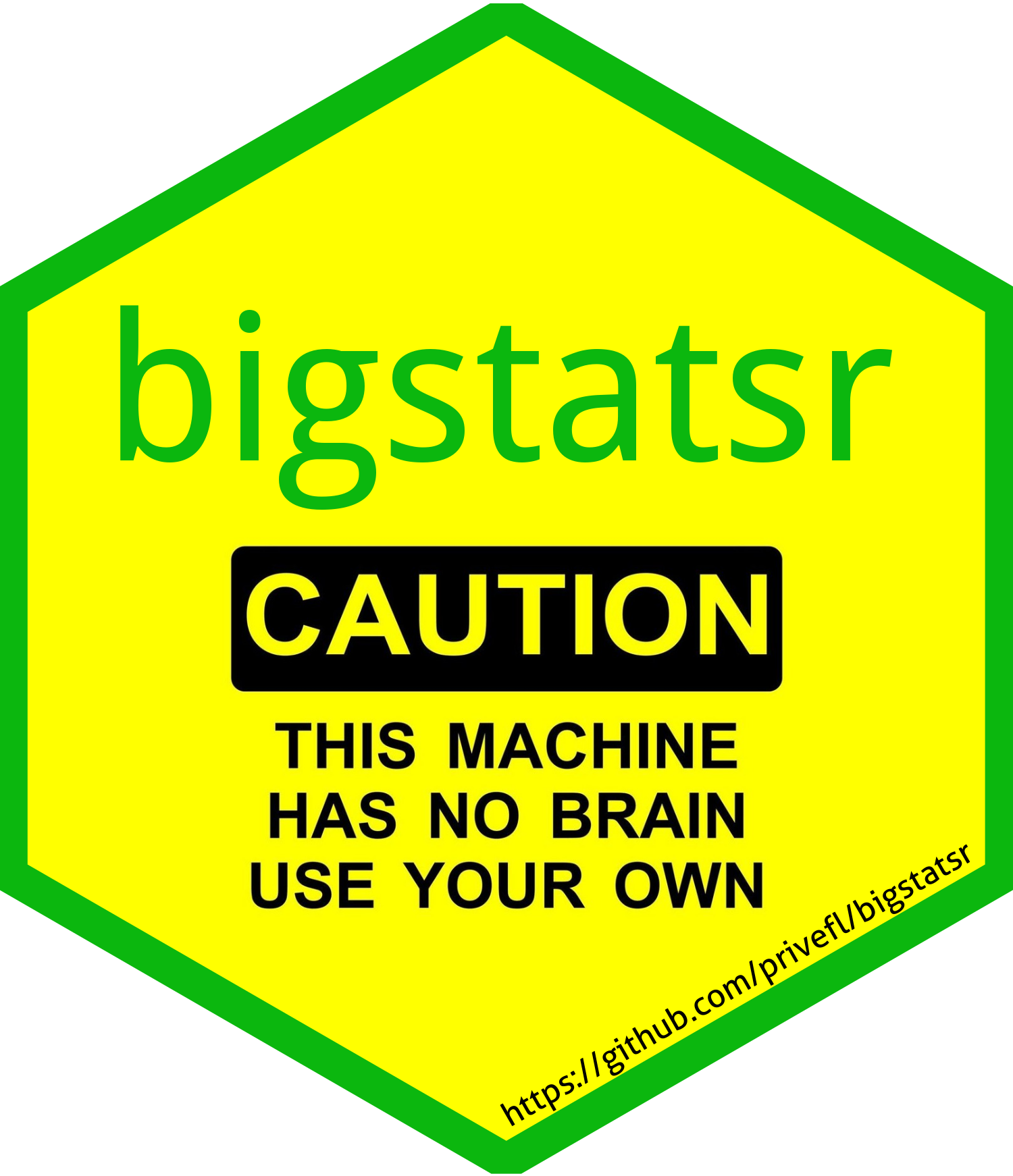R package {bigstatsr} provides functions for fast statistical analysis of large-scale data encoded as matrices. The package can handle matrices that are too large to fit in memory thanks to memory-mapping to binary files on disk. This is very similar to the format big.matrix provided by R package {bigmemory}, which is no longer used by this package (see the corresponding vignette). As inputs, package {bigstatsr} uses Filebacked Big Matrices (FBM).

LIST OF FEATURES

Note that most of the algorithms of this package don’t handle missing values.

## Installation

# For the CRAN version
install.packages("bigstatsr")
remotes::install_github("privefl/bigstatsr")

## Small example

library(bigstatsr)

# Create the data on disk
X <- FBM(5e3, 10e3, backingfile = "test")\$save()
# If you open a new session you can do
X <- big_attach("test.rds")

# Fill it by chunks with random values
U <- matrix(0, nrow(X), 5); U[] <- rnorm(length(U))
V <- matrix(0, ncol(X), 5); V[] <- rnorm(length(V))
NCORES <- nb_cores()
# X = U V^T + E
big_apply(X, a.FUN = function(X, ind, U, V) {
X[, ind] <- tcrossprod(U, V[ind, ]) + rnorm(nrow(X) * length(ind))
NULL  ## you don't want to return anything here
}, a.combine = 'c', ncores = NCORES, U = U, V = V)
# Check some values
X[1:5, 1:5]

# Compute first 10 PCs
obj.svd <- big_randomSVD(X, fun.scaling = big_scale(),
k = 10, ncores = NCORES)
plot(obj.svd)

# Cleanup
unlink(paste0("test", c(".bk", ".rds")))

If you want to use Rcpp code, look at this tutorial.

## Some use cases

### Parallelization

Package {bigstatsr} uses package {foreach} for its parallelization tasks. Learn more on parallelism with {foreach} with this tutorial.

## Bug report / Help

How to make a great R reproducible example?

Please open an issue if you find a bug.

If you want help using {bigstatsr}, please open an issue as well or post on Stack Overflow with the tag bigstatsr.

I will always redirect you to GitHub issues if you email me, so that others can benefit from our discussion.